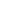# Add a pair of two-digit numbers when the ones digits have a sum less than or equal to 10.

EngageNY 60 min(s)
In this lesson, students continue adding sets of two-digit numbers, where the ones digits produce a sum less than or equal to 10. Rather than always adding tens first (as in the last lesson), they now practice adding ones to the first addend and then adding the remaining ten. For example, when adding 17 + 13, students decompose 13 into 10 and 3. Then they add 3 to 17, making 20, and add the remaining ten to find 20 + 10 = 30.